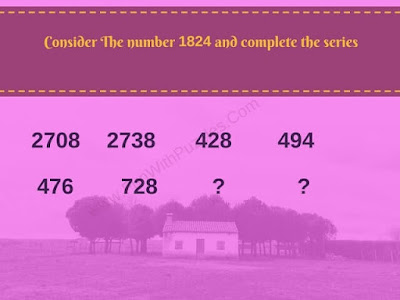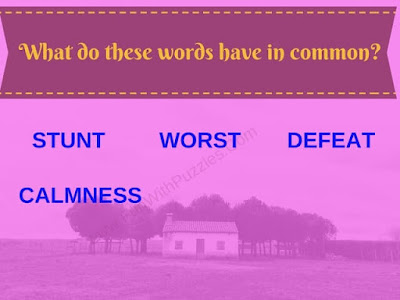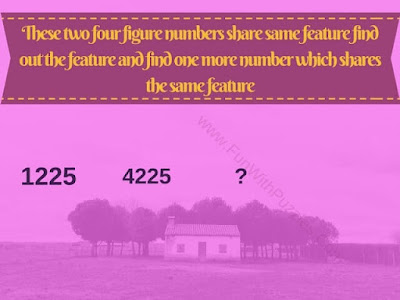There are many different brain teasers on this Fun With Puzzles website. Some are very easy brain teasers, some are medium level. However, today's brain teasers are very tough and these are not for kids or teens. Many of these brainteasers require very high thinking logical reasoning as well as mathematical calculations to find the answers to these riddles.
I am looking forward to the readers' answers to these puzzles. Let's see how many you can solve it correctly. Do post your answers in the comment section of this post.1. Can you find the missing number which will replace "?"2. Can you find the missing numbers?3. Can you fill in the missing numbers?4. What do these words have in common?5. What does this Mobile Number remind?6. Can you find the missing number?

Do checkout following mentioned Interesting yet tricky Puzzle and Brain Teasers

## List of Interesting Puzzles and Brain Teasers

1. Awesome Missing Number Math Circle Puzzles: Check out some awesome Maths Puzzles in which your challenge is to find the missing number.

2. Brain Teaser Riddles for interviews with answers: Do solve this logical reasoning and out-of-the-box thinking puzzles to get better prepared for your interviews.

3. Clever Maths Brain Teasers with answers: Another set of clever thinking Maths brain teasers to tickle your brain.

4. Brain Cracking Maths Circle Puzzles: It contains very interesting Maths Circle Puzzles which will crack your brain.

## Answers of Brain Cracking Questions

Answers to these Very Tricky Brain Cracking Questions are as given below
1.
In each row, every number describes the previous number. How many times a digit exits in the previous number.
e.g in 1225 one occurs 1 time, so it is written 11, 2 comes twice so it is written 22 and similarly 5 comes only once so it is written 15. So the answer is 112215

2.
The numbers given in this tricky brain teaser are obtained by rearranging the digits of 1824 in any order and then dividing that number by three. Most of the numbers have been already obtained by this process. The remaining two number are 716 and 947

3.
Going from top to bottom and left to right. The missing numbers are are
1, 2 and 2

4.
Every word contains three adjacent alphabets

5.
This number is the square root of two.
1.414213562

6.
The sum of the digits of each given number is 2 more than the square root of that number
12+25=37=Square root of 1225+2
4624 is the next number that shares the same feature.

----------------------------------
Here is the answer to the 14th Brain Teaser Question of post titled "Simple Math Riddles for Teens and Kids with Answers to Twist your Brain". We have hidden this answer to avoid any spoiler. Do select the text below to read the answer.
----------------------
The answer is 18. This one was very simple. Just divide the sum obtained by 2.
----------------------